## 高品质SUV引领者凯翼X5献礼宜宾优秀企业家

2019年06月11日 21:30 来源：车行天下 超过：10336次关注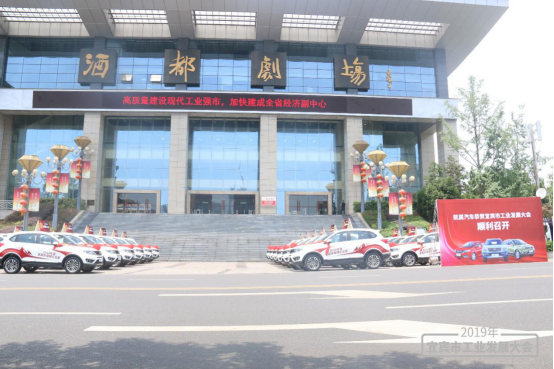6月10日，2019年宜宾工业发展大会在宜宾酒都剧场召开。本次大会以坚持“产业发展双轮驱动”战略为主题，旨在深入贯彻落实党的十九大精神，习近平新时代中国特色社会主义经济思想，围绕省政府加快构建“5+1”现代产业体系决策部署，大力实施“产业发展双轮驱动”战略，会上总结了2018年工业工作并提出2019年工业发展思路。通过表彰先进，提振信心，激发创业热情，高质量建设现代工业强市，为建成全省经济副中心提供坚强支撑。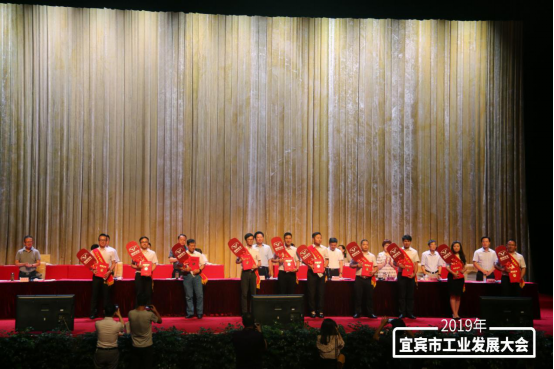凯翼汽车作为此次工业大会的战略合作伙伴，旗下凯翼X5成为此次大会优秀企业的奖励用车。市委常委，秘书长谢杰先生通报了2018年度宜宾市卓越企业家和优秀企业家评选结果，2018年度全市工业经济发展绩效评价结果等，并对评选出的企业/企业家，园区等代表进行颁奖。宜宾凯翼汽车有限公司荣获2018年宜宾造产品销售突出贡献奖及2018年度先进示范企业。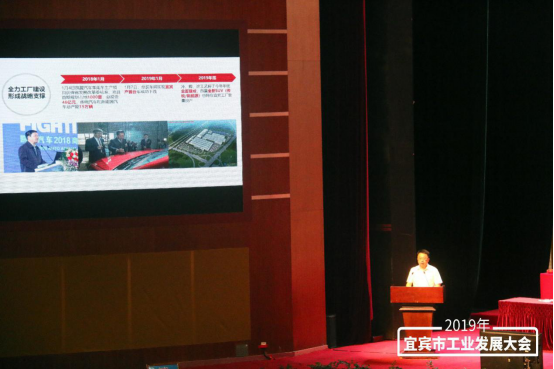据了解，宜宾凯翼汽车有限公司总经理高雷作为战略重组及市场开拓企业代表进行了发言，围绕着凯翼是如何实施战略重组，市场开拓等方面内容，与诸位领导和多位企业家进行了分享和交流。凯翼汽车展现了双轮驱动的战略思想和市场开拓的坚实步伐，赢得多方的肯定和赞赏。高总表示，“为实施巩固提升传统产业和加快发展新兴产业的“双轮驱动”战略，重点发展以新能源汽车为代表的成长型新兴产业。宜宾市经过与奇瑞汽车的积极对接，引进奇瑞汽车下属全资子公司凯翼汽车入驻宜宾，成为四川省首个拥有轿车、SUV和MPV三大系列传统和新能源车生产资质、总产能15万辆/年的整车生产企业。成为西南地区重要的综合汽车生产基地和四川省汽车产业重要一极”。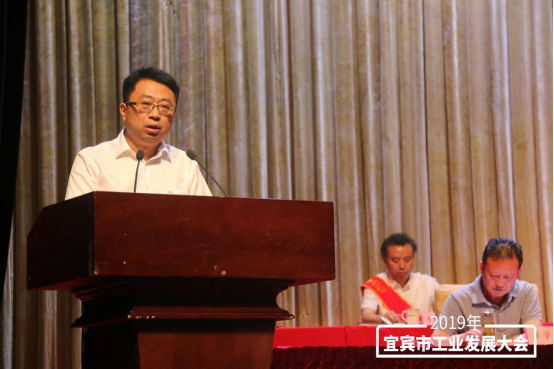为加快推进汽车产业发展，宜宾在国家级开发区临港经济技术开发区规划了5平方公里的汽车产业园及21万平米的汽车零部件配套产业园，为以凯翼汽车为主的整车生产企业提供配套服务。宜宾凯翼汽车有限公司和奇瑞汽车宜宾分公司两家乘用车整车生产项目的落户,标志着宜宾汽车产业具备了大发展的基础和平台。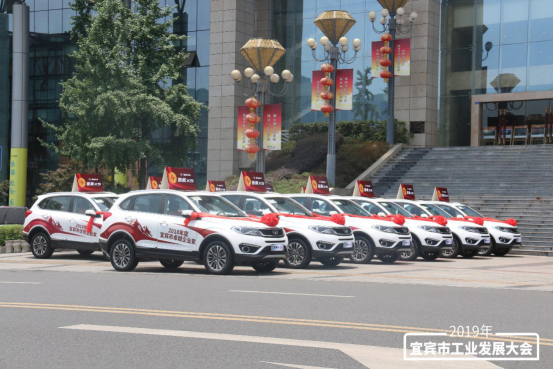2019年凯翼汽车针对品牌进行了战略性优化调整，坚持“年轻化”的品牌调性，赋予凯翼全新的品牌价值，增强用户认知。在年轻化的品牌战略下，凯翼已制定了未来五年的产品战略及规划，产品覆盖主流市场，在SUV、SD、MPV市场全面布局，同时加快产品更新迭代速度，保障产品竞争力；在新能源车型方面，早期将在传统燃油车基础上同步开发新能源车型，后期将单独规划凯翼纯电平台，打造富有凯翼特色的新能源车型。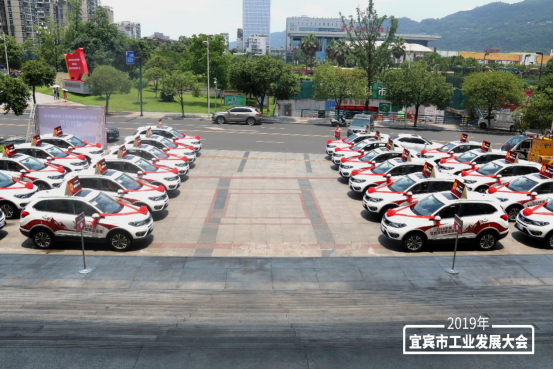凯翼汽车在新年伊始也已整体入驻成都、宜宾两地，标志着2019年品牌将进入“新凯翼”时代。随着此次公司的整体搬迁，凯翼汽车正式扎根四川，在政策、资金、场地、建设等方面，得到了宜宾市政府和相关机构的鼎力支持。而成都作为新一线城市，聚集了更多的人才、科技、物资等优势资源。将研发中心和营销中心设于成都，将为凯翼汽车未来的研发与规划提供更前沿的支持。不仅如此，凯翼汽车还希望依托这个西南进出口贸易中心，打下品牌“走出去”的基础。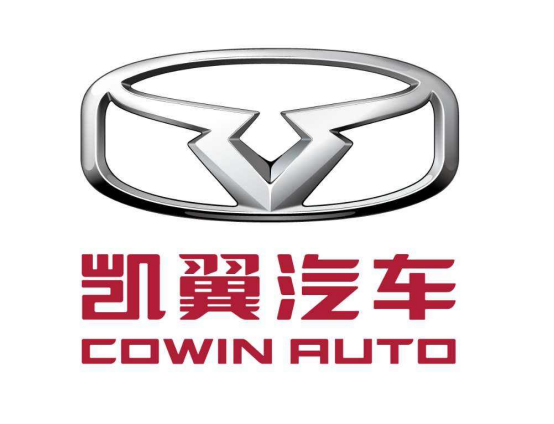对于凯翼汽车而言，2019年将开启全新的品牌变革。“结合新品牌、新工厂、新产品、新服务的“四新”规划，凯翼汽车或将在四川迎来品牌及产品的双重提升。凯翼汽车项目作为四川省、宜宾市重点项目之一，相信将助推宜宾市实施的“双轮驱动”发展目标，成为推动宜宾产业转型的重要力量。

#### 相关文章

0-500 字已有评论 0条 查看评论>>

### 热门标签

﻿
• 快速找车
• 选择品牌
• 选择品牌
• A  奥迪
• A  阿斯顿·马丁
• A  阿尔法·罗密欧
• B  宝沃
• B  布加迪
• B  巴博斯
• B  保时捷
• B  宾利
• B  奔驰
• B  宝马
• B  本田
• B  别克
• B  标致
• B  比亚迪
• B  宝骏
• B  北汽制造
• B  北汽新能源
• B  北汽幻速
• B  北汽威旺
• B  北京汽车
• B  奔腾
• B  北汽绅宝
• C  长安
• C  长安商用
• C  长城
• C  昌河
• D  大众
• D  道奇
• D  DS
• D  东南
• D  东风风神
• D  东风风行
• D  东风小康
• D  东风风度
• D  东风
• F  福特
• F  丰田
• F  菲亚特
• F  法拉利
• F  福田
• F  福迪
• F  福汽启腾
• G  观致
• G  广汽传祺
• G  广汽吉奥
• G  GMC
• H  红旗
• H  汉腾汽车
• H  哈弗
• H  哈飞
• H  海格
• H  海马
• H  华颂
• H  黄海
• H  华泰
• H  恒天
• J  吉利汽车
• J  捷豹
• J  Jeep
• J  江淮
• J  江铃
• J  金杯
• J  九龙
• J  金旅
• K  凯翼
• K  凯迪拉克
• K  克莱斯勒
• K  科尼塞克
• K  卡威
• K  开瑞
• L  路虎
• L  林肯
• L  劳斯莱斯
• L  兰博基尼
• L  雷克萨斯
• L  铃木
• L  雷诺
• L  理念
• L  力帆
• L  莲花汽车
• L  猎豹
• L  路特斯
• L  陆风
• M  马自达
• M  MG
• M  MINI
• M  玛莎拉蒂
• M  摩根
• M  迈凯轮
• N  纳智捷
• O  欧宝
• O  讴歌
• O  欧朗
• Q  奇瑞
• Q  起亚
• Q  启辰
• R  日产
• R  荣威
• R  瑞麒
• S  三菱
• S  斯威汽车
• S  萨博
• S  smart
• S  斯柯达
• S  斯巴鲁
• S  思铭
• S  双龙
• S  上汽大通
• S  双环
• T  特斯拉
• T  腾势
• W  沃尔沃
• W  五菱汽车
• W  五十铃
• W  威兹曼
• W  威麟
• X  现代
• X  雪佛兰
• X  雪铁龙
• X  西雅特
• Y  一汽
• Y  英菲尼迪
• Y  英致
• Y  依维柯
• Y  野马汽车
• Y  永源
• Z  众泰
• Z  中华
• Z  中兴
• Z  知豆
• 选择车系
• 选择车系
• 车型对比
• 选择品牌
• 选择品牌
• A  奥迪
• A  阿斯顿·马丁
• A  阿尔法·罗密欧
• B  宝沃
• B  布加迪
• B  巴博斯
• B  保时捷
• B  宾利
• B  奔驰
• B  宝马
• B  本田
• B  别克
• B  标致
• B  比亚迪
• B  宝骏
• B  北汽制造
• B  北汽新能源
• B  北汽幻速
• B  北汽威旺
• B  北京汽车
• B  奔腾
• B  北汽绅宝
• C  长安
• C  长安商用
• C  长城
• C  昌河
• D  大众
• D  道奇
• D  DS
• D  东南
• D  东风风神
• D  东风风行
• D  东风小康
• D  东风风度
• D  东风
• F  福特
• F  丰田
• F  菲亚特
• F  法拉利
• F  福田
• F  福迪
• F  福汽启腾
• G  观致
• G  广汽传祺
• G  广汽吉奥
• G  GMC
• H  红旗
• H  汉腾汽车
• H  哈弗
• H  哈飞
• H  海格
• H  海马
• H  华颂
• H  黄海
• H  华泰
• H  恒天
• J  吉利汽车
• J  捷豹
• J  Jeep
• J  江淮
• J  江铃
• J  金杯
• J  九龙
• J  金旅
• K  凯翼
• K  凯迪拉克
• K  克莱斯勒
• K  科尼塞克
• K  卡威
• K  开瑞
• L  路虎
• L  林肯
• L  劳斯莱斯
• L  兰博基尼
• L  雷克萨斯
• L  铃木
• L  雷诺
• L  理念
• L  力帆
• L  莲花汽车
• L  猎豹
• L  路特斯
• L  陆风
• M  马自达
• M  MG
• M  MINI
• M  玛莎拉蒂
• M  摩根
• M  迈凯轮
• N  纳智捷
• O  欧宝
• O  讴歌
• O  欧朗
• Q  奇瑞
• Q  起亚
• Q  启辰
• R  日产
• R  荣威
• R  瑞麒
• S  三菱
• S  斯威汽车
• S  萨博
• S  smart
• S  斯柯达
• S  斯巴鲁
• S  思铭
• S  双龙
• S  上汽大通
• S  双环
• T  特斯拉
• T  腾势
• W  沃尔沃
• W  五菱汽车
• W  五十铃
• W  威兹曼
• W  威麟
• X  现代
• X  雪佛兰
• X  雪铁龙
• X  西雅特
• Y  一汽
• Y  英菲尼迪
• Y  英致
• Y  依维柯
• Y  野马汽车
• Y  永源
• Z  众泰
• Z  中华
• Z  中兴
• Z  知豆
• 选择车系
• 选择车系
• 选择车型
• 选择车型
• 意见反馈